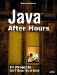# Creating the Fish

 Each Fish object has three methodsa constructor, a swim method that moves the fish, and a drawImage method that draws the fish using the Graphics object you pass it. The constructor's job is to store what's passed to itthe two fish images, the bounding rectangle of the aquarium, and the object that corresponds to the tank itself. In addition, the constructor creates a random number generator seeded with the current system time in milliseconds (returned by the System.currentTimeMillis method), and it uses this random number generator to create a random location and velocity for the fish. After the random number generator is seeded, every time you call its nextInt method, you'll get a new, random integer from 0 to 232. Both the fish's location and velocity are stored in Java Point objects, which have x and y members; all values are stored in pixels. The location variable holds the position of the upper-left corner of the fish image, and the velocity variable holds the X and Y number of pixels that will be added to the fish's location each time the swim method is called. Here's what the Fish class's constructor looks like: ` class Fish { Component tank; Image image1; Image image2; Point location; Point velocity; Rectangle edges; Random random; public Fish(Image image1, Image image2, Rectangle edges, Component tank) { random = new Random(System.currentTimeMillis()); this.tank = tank; this.image1 = image1; this.image2 = image2; this.edges = edges; this.location = new Point(100 + (Math.abs(random.nextInt()) % 300), 100 + (Math.abs(100 + random.nextInt()) % 100)); this.velocity = new Point(random.nextInt() % 8, random.nextInt() % 8); } . . . } ` That initializes the fish; how about making them do something?Java After Hours: 10 Projects Youll Never Do at Work
ISBN: 0672327473
EAN: 2147483647
Year: 2006
Pages: 128